Engineering Jobs   »   GATE 2023   »   GATE 2023 Memory Based Question Paper

# GATE 2023 Memory Based Question Papers of CS, ME, EE, CE, EC, IN

GATE 2023 Memory Based Question Paper: GATE 2023 was held successfully on the 4th, 5th, 11th, and 12th of February 2023 at the 219 examination centres. The candidates who have taken the GATE 2023 Exam can get the branch-wise GATE 2023 Question Paper through this article.

## GATE 2023 Memory Based Question Paper

IIT Kanpur hosted the Graduate Aptitude Test in Engineering (GATE) Exam 2023 for aspirants willing to pursue a career in the reputed PSU, further studies from various IITs, NITs, etc. on the 4th, 5th, 11th, and 12th of February 2023. The GATE Authority will release the GATE 2023 Answer Key on 21 February 2023 on its official website. Meanwhile, the aspirants can check the GATE 2023 Memory Based Question Paper covered in this article for analyzing their questions paper.

## GATE 2023 Memory Based Question Paper PDF

The GATE 2023 Examination was held on the 4th, 5th, 11th, and 12th of February 2023 in the forenoon and afternoon session for 29 disciplines across India. The aspirants who have appeared in the GATE 2023 Exam are thinking about several questions like how was the paper, and whether the correct answer was marked by them.

Here, is the answer to all your questions framed by the ADDA247 team in the form of the GATE 2023 Memory Based Question Paper. We have consolidated here complete details regarding GATE 2023 Memory Based Question Paper for the ease of candidates. Also, bookmark this website for further Engineering Job Updates.

## GATE 2023 Exam Overview

The Memory Based Questions are provided after the GATE Exam gets over. GATE 2023 Memory Based Questions will help the candidates to calculate the GATE Score after analyzing the GATE 2023 Exam. Candidates who are going to take GATE 2024 Exam can prepare through the questions provided here.

 GATE 2023 Exam Overview Exam Name Graduate Aptitude Test in Engineering Conducting Organization IIT Kanpur Online Registration Start 30th August 2022 Last date to Apply (With Late Fees) 07th October 2022 Category Engineering Jobs GATE Admit Card 2023 Date 9th January 2023 GATE 2023 Exam Dates 4, 5, 11, 12 February 2023 GATE 2023 Answer Key To Be Out on 21 February 2023 GATE 2023 Official Website https://gate.iitk.ac.in/

The GATE 2023 consisted of questions from general aptitude, engineering mathematics, and technical section. The candidates who have appeared in the GATE 2023 Exam can check the branch-wise memory-based GATE 2023 Question Paper through this article.

Candidates who appeared for GATE 2023 Exam conducted on 4th, 5th, 11th, and 12th Feb 2023 can be able to download the GATE 2023 Memory Based Questions asked in the Exam. Here, we are providing the GATE 2023 Memory Based Questions Pdf for the candidates to analyze the difficulty level of the questions asked in the GATE Examination. Click the links below to download GATE Memory Based Questions Pdf.

 GATE 2023 Memory Based Questions Pdf GATE 2023 ME Memory Based Questions Download PDF GATE 2023 EE Memory Based Questions Download PDF GATE 2023 ECE Memory Based Questions Download PDF GATE 2023 CE Memory Based Questions (Morning Shift) Download PDF GATE 2023 CE Memory Based Questions (Evening Shift) Download PDF GATE 2023 IN Memory Based Questions Will Be Available Soon GATE 2023 CSE Memory Based Questions Will Be Available Soon

## Memory Based GATE 2023 Question Paper CS

The GATE 2023 Exam was held successfully on 4th February 2023 first shift (9:30 am to 12:30 pm) for Computer Science Engineering. The aspirants who have taken the exam shared the following memory-based questions:

Q.1.Minimum number of states in DFA which do not accept  state.

Q.2. Pipeline of stage 3 with times 10,20,14 find time for execution of 100 instructions no stalls are involved

Q.3. Calculate the tag bits, the cache size is 64kb and the cache is 8-way set associative, system address is 32-bit.

Q.4. Total page 30, 1 page =512 entries and its array and elements are accessed using for loop to find the number of page faults.

Q.5. Problem on Degree of relation.

Q.6. (132)4=(  )5

Q.7. Questions based on triple integration.

Q.8. Maxima Minima Problem(MSQ)

F(x)=x3 +15x2-33x-36

Q.10. Find out the relation between f(n)=n and g(n)=n2

## GATE 2023 Exam Mechanical Engineering Memory Based Questions

The GATE 2023 Exam has been successfully conducted in the afternoon session (02:30 PM to 05:30 PM) for Mechanical Engineering. The candidates who appeared in the GATE ME Exam 2023 are now looking to check the questions which were asked in the exam to analyze their performance. ADDA247 Team is providing a brief analysis for GATE 2023 Exam to help the aspirants. Candidates can check the questions shared by students with us in the below section.

## Memory Based GATE 2023 Question Paper ME

The candidates who appeared in the GATE ME Paper held on 04th February 2023 can check the questions which were asked in the exam. The list of questions shared by aspirants are listed below:

Q.1 Planting: Seeds : : Raising : _____

Q.2 He did not manage to fix the car himself, so he ___ in the garage.

Q.3 One Question on Complex Function.

Q.4 How many pair of sets (S, T) are possible among the subsets of (1, 2, 3, 4, 5, 6) that satisfy the conditions that S is a subset of T?

Q.5 A cylindrical transmission shaft of length 1.5 m and diameter 100 mm is made of a linear elastic material with a shear modulus of 80 GPa. While operating at 500 rpm, the angle of twist across its length is found to be 0.5 degree. Find the power transmitted by the shaft at this speed ____ kW. (Rounded off two decimal places) Take π = 3.14.

Q.6 Two meshing spur gears and 2 with diametric pitch of 8 teeth per mm and an angular velocity w2/w1 = 1/4 have their centres 30 mm apart. The number of teeth on the driver gear (gear 1) is ___.

Q.7 A machine produces defective component with probability of 0.015 and total defective components is 200. It follows poisson distribution then find the value of lambda.

Q.8 Two surfaces P and Q joined together with no melting of both the surfaces creating the joints of which welding operation?

Q.9 EOQ Model statement based question.

Q.10 The atomic radius of hypothetical FCC Model is √2/10 nm. The atomic weight of material is 24.092 g/mol. Find the density of metal by considering the Avagadro’s number 6.023*10²³

Q.11 The effective stiffness of a cantilever beam of length L and flexural rigidity EI subjected to transverse load W is___

Q.12 Consider a counter flow heat exchanger with the inlet temperature of two fluids (a & b) being Ta = 300K, and Tb = 350K. The heat capacity rates of both the fluids are Ca = 1000W/K and Cb = 400 W/K and the effectiveness is 0.5. The actual heat transfer rate in kW is ____.

Q.13 A cylindrical rod of length l and diameter d is placed inside a cube enclosed of side length L. The inner surface of the cube is denoted by S. The view factor will be ___

Q.14 SN Curve based numerical.

Q.15 Consider an isentropic flow of air (Y = 1.4) through a duct. The variation in the flow across the section are negligible. The flow condition at end 1 is as follows:

P1 = 100 kPa, S1 = 1.2kg/m, u1 = 400 m/s.  The cross-sectional area of the duct at end 2 is given by A2 = 2A1, where A denotes the area of cross-section at the end 1. What will be the true condition for the velocity u2 and pressure P2 at the end 2 is ____.

Q.16 A steel plate with 1.5 wt % carbon is slowly cooled from 1100 C just below its eutectoid temperature (723 C). The ration of the pro-eutectoid cementite content in the microstructure that develops just below eutectoid temperature is ___

Q.17 Consider the second order linear differential equation x2 d2y/dx2 + xdy/dx – y = 0, x>=1 with the condition y (x=1) = 6, dy/dx = 2. Find the value of y at x=2.

Q.18 The smallest perimeter that a rectangle with area of 4 sq. units can have ____.

## GATE 2023 Exam Electrical Engineering Memory Based Questions

The GATE 2023 Electrical Engineering Exam was held on 5th February 2023 Forenoon Session (FN) i.e. 09:30 am to 12:30 pm. The aspirants who have taken the exam share some of the questions that they were able to recall. The candidates can check the GATE 2023 EE Memory based questions given below:

Q.1.Find out Vab for the following: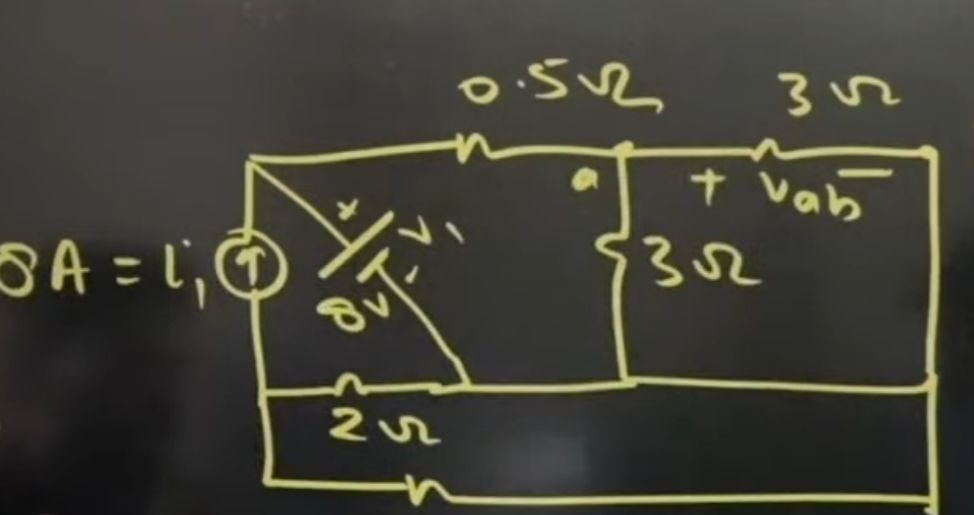Q.2. Find out the voltage at the inductor at t=0+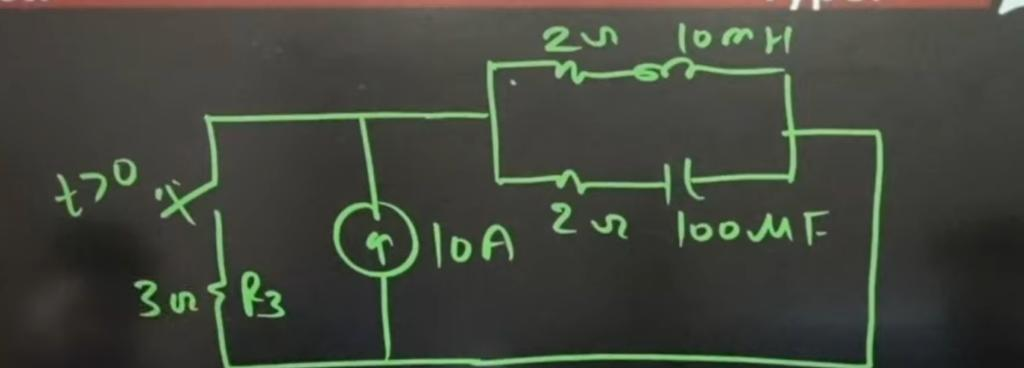Q.3. 10 poles, 50 Hz 240 KV 1 phase induction motor rums at 540 rpms. Find out the frequency of the induced current.

Q.4.Find out Vo=?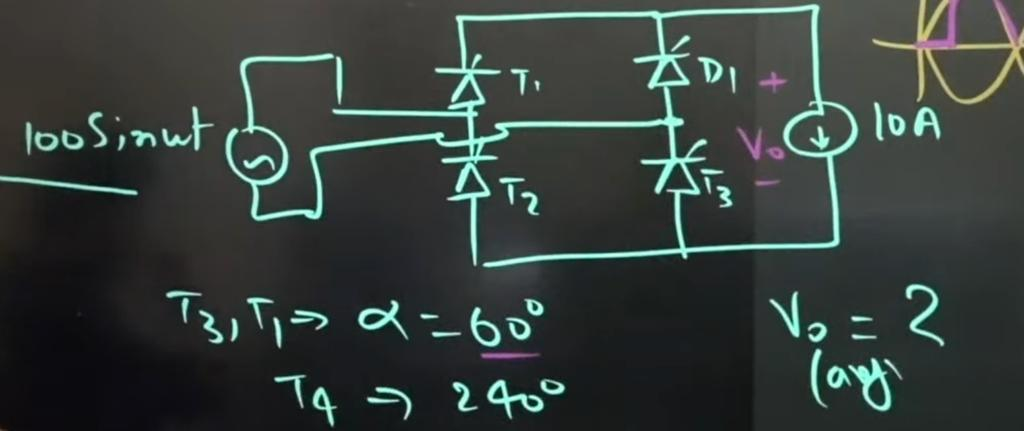Q.5. For a given vector W=[ 1 2 3]T Find out the vector normal to the pole defined by WTX=1.

Q.6. Find out Vo max and min for the circuit given below: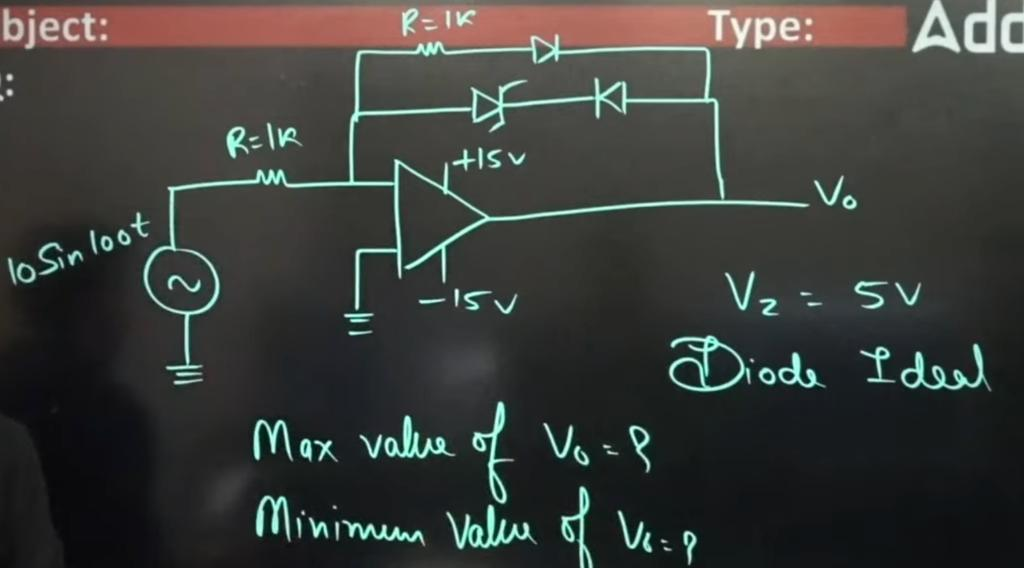Q.7. For a separately excited DC motor rated 400v, 15 A, 1500 rpm, constant torque load at rated speed operating frequency from 400 V DC supply Rq=1.2 ohm. If the supply voltage drops by 10%, Find out the New speed in rpm.

Q.8. Neglecting the delays. Find out the decimal equivalent of ABCD which will not change with a clock for the circuit given below: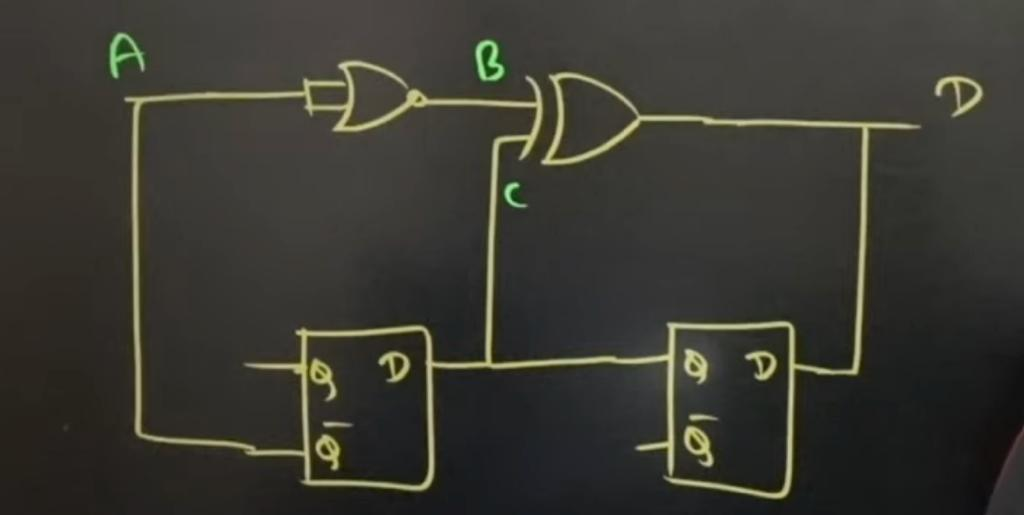Q.9. For the circuit given below find out Vth at t=5 msec.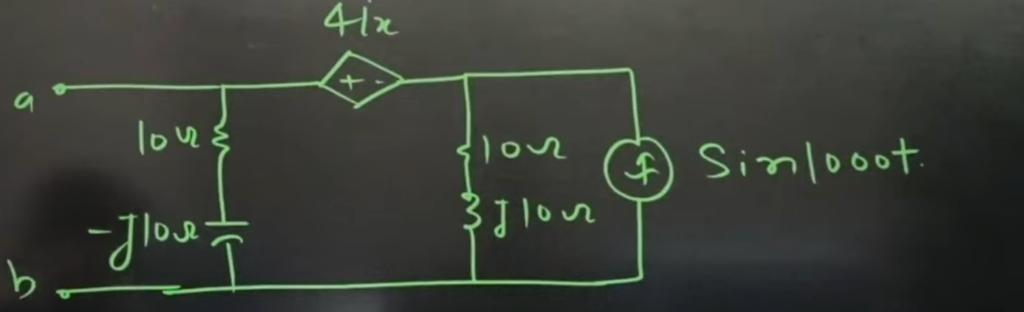## GATE 2023 ECE Exam Memory Based Questions

GATE 2023 Exam for Electronics Engineering Branch Candidates has been conducted on 5th February 2023. As the exam gets over, Students are now searching for the Questions that were asked in the GATE ECE 2023 Exam. Here, we are providing the GATE 2023 Memory Based Questions for Electronics Engineering. Click on the download link provided in the article above to download the GATE ECE Questions.

## GATE 2023 IN Exam Memory Based Questions

The GATE Exam 2023 for Instrumentation Engineering has been conducted on 11th February 2023 in the forenoon session(09:30 AM to 12:30 PM). The candidates who appeared in the GATE IN Paper 2023 shared the memory-based questions with us. Based on the student’s feedback we have provided a list of questions asked in the GATE Instrumentation Exam 2023 discussed below. Candidates must refer below to check the GATE 2023 IN Memory Based Questions.

Q.1 What will be the next term 1, 3, 5, 7, 9, ___.

Q.2 An opaque cylinder is kept in contact with a horizontal beam of light then the shape will shown on the application of light.

Q.3 Evaluate x. sin(1/x) with lim x →0

Q.4 x¨= – Kx·{x·(0) = 1, x·(0) = 0} then select the correct statement.

Q.5 Consider the real valued function g(x) = (x – 2)² – 2x + 7 with (-∞, ∞) then find the minimum value of g(x)?

Q.6 Matrix-based question.

Q.7 One question from Standard Deviation.

Q.8 Function F(z) = 1/z is expanded up to ∞ power series around (z=2) would result in F(z) = ∑ak (z-2) to power k. Find the best suitable option.

Q.9 y(s)/x(s) = s – π/s + π and y(t) = sinπ t, then find x(t)=?

Q.10 h(s) = δt + 0.5δ (t – 4) & x(t) = cos (7π/4t), Find the output.

## GATE 2023 February 12 Shift 1 Civil Engineering Question Paper

IIT Kanpur conducted the GATE 2023 CE Paper on 12th February 2023. Now the candidates who appeared in this exam are now searching for the questions asked in the exam. The students who have taken the GATE CE Exam 2023 shared the memory-based questions with our team. We have listed all the memory-based questions of the GATE Civil Paper here to help the aspirants. So, check all the questions given below to understand the level of the question paper.

Q. Eject : Insert : : Advance : _______ (Retreat)

Q. I have not yet decided, what will I do this evening? I ______ visit a friend. (might)

Q. Name the water borne diseases caused by viral pathogens.

Q. dy/dx + α.xy = 0 with boundary conditions y = 1 at x = 0, and y = 8 at x = 1 then find the value of α.

Q. Solve the integral I = ∫ 1/x² dx

Q. P(A) = 0.5 & P(B) = 0.8 and P(a) = 0.5 & P(b) = 0.2. Find the probability of at least one happening.

Q. Given data S = 50%, G = 2.6, w = 15% then find the value of e. (Formula Se = wG)

Q. Transition Curve, R = 300m, V = 15m/sec, C = 0.75 m.sec³, Ls =? (Formula Ls = V³/C.R)

Q. A drained direct shear test is carried out on a specimen under a normal stress of 80kfg. The specimen gets failed at a shear stress of 35 KPa. The angle of internal friction will be.

Q. Compaction Curve based statement question.

Q. Aw = 100, B = 120d, Δt = 35cm, Kp = 30d, Kd = 12cm.

Q. Water water inflow in ASP = 0.5m³/sec and F/m ratio = 0.20 mg/mg day. Influent biodegradable organic matter (after primary setting) is 150mg/l and MLVSS = 2000mg/l complete removal of biodegradable organic matter. Find the volume of irration time?

Q. Given σ = 50KPa, τ = 35KPa, Φ = ?

## GATE 2023 February 12 Shift 2 Civil Engineering Question Paper

The candidates who appeared in the GATE 2023 Civil Engineering Examination shared some of the questions with us after the examination was over. Engineers Adda team has consolidated all of the possible questions for the sake of ease of candidates. The GATE 2023 CE memory-based questions are given below:

Q. When Elevation and Temperature are given. Find out the Lapse rate in(oc /100m)

 Elevation Temperature 5 m 14.2 325 m 16.9

Q.SSD is equal to (a) Breaking Distance (b)Breaking Distance + Distance Traveled during reaction time (c)Breaking Distance + Perception reaction time (d)Distance Traveled during reaction time

Q.Given: D=0.6m, L=8m, r=19KN/m, c=25 KPa, FOS=3, Nc =9 then find out the safe load or allowable load. Given that alpha is 1.

Q.Match the following

 I II Horton’s Equation Muskingum Equation Penman Equation Precipitation Flood Frequency Evapotranspiration Infiltration Channel Routing

Q.(48)^x=256 then find out x.

Q. Which of the following are not active disinfectants?

1. Cl
2. O3
3. OCl
4. OH

Q.Kind:_______::often: seldom

1. Cruel
2. Kindness
3. Variety
4. Type

Q.Match the following

 I II Aromatic Hydrocarbon Carbon mono oxide Sulphur Oxide Ozone Reduce the capacity of blood to carry oxygen Bronchitis on pulmonary Damages Chromosomes Carcinogenic Effect

Q. As per IRC Guidelines( IRC 86: 2018) Extra widening depends on which of the follwing parameter?

1. number of lanes
3. S.E.

Q.For an unconfined compression test, σ3=0, σd=76 KPa. Find c.

Q.For the given vector space [2 1 0 3]T and [1 0 1 2]T belongs to null space then find the other vector belonging to null space.

1. [0 -2 1 -1]T
2. [1 1 -1 1]T
3. [2 0 1 2]T
4. [3 1 1 2]T

Q. Magnetic Bearing= 183o30′(noon, sun) then find Magnetic Declination.

Q. If the size of the ground area is 6 km x 3 km and the corresponding photograph size is 3 cm x 15 cm. then find out Scale 1:?

Q. In a 3*3 grid, Find out the number of shaded so that only one shaded block will be there in a row and column.

Q. For the given figure find out σx and σy.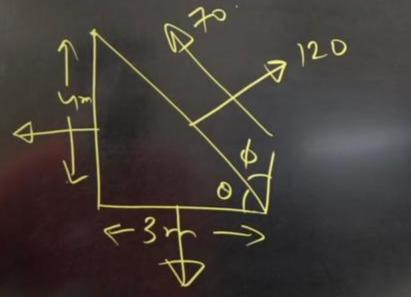Q. Number of independent Eigen Vector of the given 3*3 matrix.

Q. A line ran_________ page.

Q. A round table has 6 identical chairs then find out the number of possible ways that couples sit together(there are 3 married couples).

Q. In the context of water and wastewater treatment the correct statement is?

1. ammonia decreases chlorine demand
2. Phosphorous stimulates algae and aquatic growth
3. Ca and Mg increase hardness and TDS
4. Particulate matter may shield microorganisms during disinfection

Now keep yourself updated with Latest Engineering Government Jobs 24×7 Download ADDA247 App Now! CLICK HERESharing is caring!

## FAQs

### How to check GATE 2023 Memory Based Question Paper?

The candidates can check the GATE 2023 Memory Based Question Paper through this article.

### When was the GATE 2023 Exam held?

The GATE 2023 Exam was held on the 4th, 5th, 11th, and 12th of February.

### When will the GATE 2023 response sheet be released?

The GATE 2023 response sheet will be released on 15th February 2023.

### When will the GATE 2023 Result released?

As per the exam schedule, IIT Kanpur will release the GATE 2023 Result on 16th March 2023.

### TOPICS:

•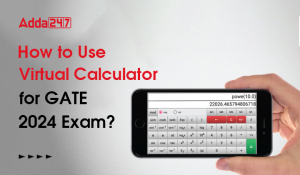How to Use Virtual Calculator for GATE 2...
•PSU Recruitment Through GATE 2023, Check...
•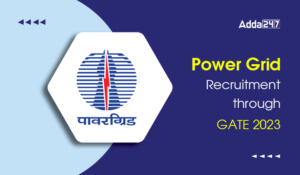Power Grid Recruitment Through GATE 2023...
•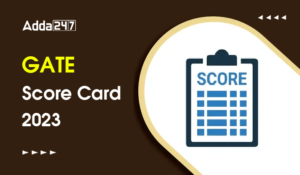GATE Score Card 2023 Out, Direct Link to...
•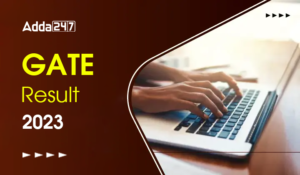GATE Result 2023 Out, Download Result, C...
•Benefits of GATE Exam, Find Importance o...